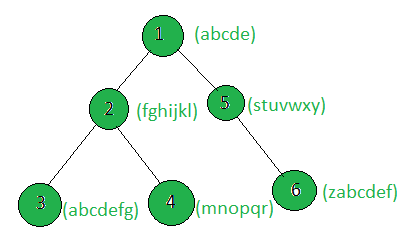# Count the nodes of the tree which make a pangram when concatenated with the sub-tree nodes

Given a tree, and the weights (in the form of strings) of all the nodes, the task is to count the nodes whose weighted string when concatenated with the strings of the sub-tree nodes becomes a pangram.
Pangram: A pangram is a sentence containing every letter of the English Alphabet.

Examples:

Input:Output: 1
Only the weighted string of sub-tree of node 1 makes the pangram.

## Recommended: Please try your approach on {IDE} first, before moving on to the solution.

Approach: Perform dfs on the tree and update the weight of every node such that it stores its weight concatenated with the weights of the sub-tree nodes. Then, count the nodes whose updated weighted string forms a pangram.

Below is the implementation of the above approach:

## C++

 `// C++ implementation of the approach ` `#include ` `using` `namespace` `std; ` ` `  `vector<``int``> graph; ` `vector weight(100); ` ` `  `// Function that returns if the ` `// string x is a pangram ` `bool` `Pangram(string x) ` `{ ` `    ``map<``char``, ``int``> mp; ` `    ``int` `n = x.size(); ` ` `  `    ``for` `(``int` `i = 0; i < n; i++) ` `        ``mp[x[i]]++; ` `    ``if` `(mp.size() == 26) ` `        ``return` `true``; ` `    ``else` `        ``return` `false``; ` `} ` ` `  `// Function to return the count of nodes ` `// which make pangram with the ` `// sub-tree nodes ` `int` `countTotalPangram(``int` `n) ` `{ ` `    ``int` `cnt = 0; ` `    ``for` `(``int` `i = 1; i <= n; i++) ` `        ``if` `(Pangram(weight[i])) ` `            ``cnt++; ` `    ``return` `cnt; ` `} ` ` `  `// Function to perform dfs and update the nodes ` `// such that weight[i] will store the weight[i] ` `// concatenated with the weights of ` `// all the nodes in the sub-tree ` `void` `dfs(``int` `node, ``int` `parent) ` `{ ` ` `  `    ``for` `(``int` `to : graph[node]) { ` `        ``if` `(to == parent) ` `            ``continue``; ` `        ``dfs(to, node); ` `        ``weight[node] += weight[to]; ` `    ``} ` `} ` ` `  `// Driver code ` `int` `main() ` `{ ` `    ``int` `n = 6; ` ` `  `    ``// Weights of the nodes ` `    ``weight = ``"abcde"``; ` `    ``weight = ``"fghijkl"``; ` `    ``weight = ``"abcdefg"``; ` `    ``weight = ``"mnopqr"``; ` `    ``weight = ``"stuvwxy"``; ` `    ``weight = ``"zabcdef"``; ` ` `  `    ``// Edges of the tree ` `    ``graph.push_back(2); ` `    ``graph.push_back(3); ` `    ``graph.push_back(4); ` `    ``graph.push_back(5); ` `    ``graph.push_back(6); ` ` `  `    ``dfs(1, 1); ` ` `  `    ``cout << countTotalPangram(n); ` ` `  `    ``return` `0; ` `} `

## Python3

 `# Python3 implementation of the approach ` `graph ``=` `[[] ``for` `i ``in` `range``(``100``)] ` `weight ``=` `[``0``] ``*` `100` ` `  `# Function that returns if the ` `# string x is a pangram ` `def` `Pangram(x): ` `    ``mp ``=` `{} ` `    ``n ``=` `len``(x) ` `    ``for` `i ``in` `range``(n): ` `        ``if` `x[i] ``not` `in` `mp: ` `            ``mp[x[i]] ``=` `0` `        ``mp[x[i]] ``+``=` `1` `    ``if` `(``len``(mp)``=``=` `26``): ` `        ``return` `True` `    ``else``: ` `        ``return` `False` ` `  `# Function to return the count of nodes ` `# which make pangram with the ` `# sub-tree nodes ` `def` `countTotalPangram(n): ` `    ``cnt ``=` `0` `    ``for` `i ``in` `range``(``1``, n ``+` `1``): ` `        ``if` `(Pangram(weight[i])): ` `            ``cnt ``+``=` `1` `    ``return` `cnt ` ` `  `# Function to perform dfs and update the nodes ` `# such that weight[i] will store the weight[i] ` `# concatenated with the weights of ` `# all the nodes in the sub-tree ` `def` `dfs(node, parent): ` `    ``for` `to ``in` `graph[node]: ` `        ``if` `(to ``=``=` `parent): ` `            ``continue` `        ``dfs(to, node) ` `        ``weight[node] ``+``=` `weight[to] ` ` `  `# Driver code ` `n ``=` `6` ` `  `# Weights of the nodes ` `weight[``1``] ``=` `"abcde"` `weight[``2``] ``=` `"fghijkl"` `weight[``3``] ``=` `"abcdefg"` `weight[``4``] ``=` `"mnopqr"` `weight[``5``] ``=` `"stuvwxy"` `weight[``6``] ``=` `"zabcdef"` ` `  `# Edges of the tree ` `graph[``1``].append(``2``) ` `graph[``2``].append(``3``) ` `graph[``2``].append(``4``) ` `graph[``1``].append(``5``) ` `graph[``5``].append(``6``) ` ` `  `dfs(``1``, ``1``) ` `print``(countTotalPangram(n)) ` ` `  `# This code is contributed by SHUBHAMSINGH10 `

Output:

```1
```

Attention reader! Don’t stop learning now. Get hold of all the important DSA concepts with the DSA Self Paced Course at a student-friendly price and become industry ready.

My Personal Notes arrow_drop_upCheck out this Author's contributed articles.

If you like GeeksforGeeks and would like to contribute, you can also write an article using contribute.geeksforgeeks.org or mail your article to contribute@geeksforgeeks.org. See your article appearing on the GeeksforGeeks main page and help other Geeks.

Please Improve this article if you find anything incorrect by clicking on the "Improve Article" button below.

Improved By : SHUBHAMSINGH10